JEE  >  Molecular Orbital Theory

# Molecular Orbital Theory Notes | Study Chemistry for JEE - JEE

## Document Description: Molecular Orbital Theory for JEE 2022 is part of Chemical Bonding and Molecular Structure for Chemistry for JEE preparation. The notes and questions for Molecular Orbital Theory have been prepared according to the JEE exam syllabus. Information about Molecular Orbital Theory covers topics like Fajan's Rule: and Molecular Orbital Theory Example, for JEE 2022 Exam. Find important definitions, questions, notes, meanings, examples, exercises and tests below for Molecular Orbital Theory.

Introduction of Molecular Orbital Theory in English is available as part of our Chemistry for JEE for JEE & Molecular Orbital Theory in Hindi for Chemistry for JEE course. Download more important topics related with Chemical Bonding and Molecular Structure, notes, lectures and mock test series for JEE Exam by signing up for free. JEE: Molecular Orbital Theory Notes | Study Chemistry for JEE - JEE
 1 Crore+ students have signed up on EduRev. Have you?

• The Molecular Orbital Theory (often abbreviated to MOT) is a theory on chemical bonding developed at the beginning of the twentieth century by F. Hund and R. S. Mulliken to describe the structure and properties of different molecules.
• The valence-bond theory failed to adequately explain how certain molecules contain two or more equivalent bonds whose bond orders lie between that of a single bond and that of a double bond, such as the bonds in resonance-stabilized molecules.
• The key features of the molecular orbital theory are listed below.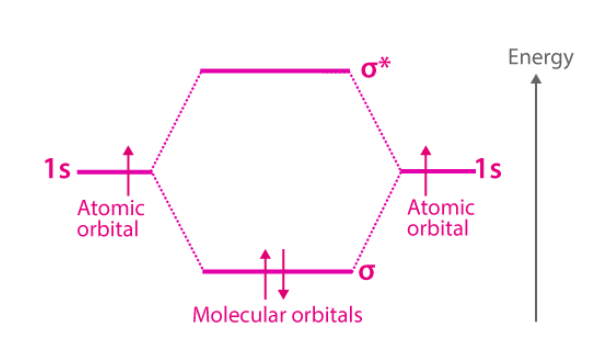(I) There is Linear combination of Atomic Orbitals (LCAO) to form molecular orbitals (M.O.)

(II) The number of molecular orbitals produced is equal to the number of atomic orbitals that are combined.

(III) Two types of molecular orbitals are formed.

(a) Bonding molecular orbitals at a lower energy than the combining atomic orbitals which favour bond formation.

(b) Anti - bonding molecular orbitals at a higher energy than the combining atomic orbitals which opposes the bond formation.

Following molecular orbitals are formed when atomic orbitals overlap.

 Atomic orbitals Bonding M. O. Anti-bonding M.O. 1s + 1s σ1s σ* 1s 2s + 2s σ2s σ* 2s 2px + 2px σ 2px σ* 2px 2py + 2py π 2py π* 2py 2pz + 2pz π 2pz π* 2pz

Electrons are filled in the increasing order of energy of the molecular orbitals which is in order of Aufbau rule

(a) When total electrons in the molecule or ions are more than 14(simple order of energy level of M. O.).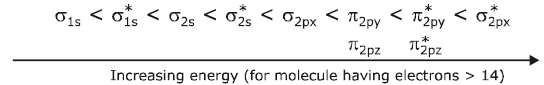(b) When total number of electrons in the molecule are ions are  ≤ 14 (modified order of energy level of M.O.).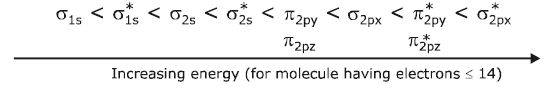(I) The electrons are filled in molecular orbitals in the same way as in atomic orbitals, that is, as per the Hund's Rule, Pauli exclusion principle and Aufbau rule.

(II) Number of covalent bonds between two atoms is called Bond order and is given by Bond order (B.O.) =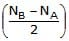Where, NB = number of electrons in bonding molecular orbital and NA = number of electrons in anti bonding molecular orbitals.

(III) For a stable molecule/ion, NB > NA

(IV) A given molecule or ion is paramagnetic if it has unpaired electrons in the molecular orbital. Otherwise it is diamagnetic

(V) Greater the bond order, shorter the bond length and greater the bond energy. Thus for O2, O2  , O2,O22-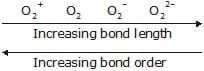Conditions for atomic orbitals to form M.O.

• The combining A.O. should be of comparable energy.
• The combining atomic orbitals must overlap to a large extent greater the overlap, stable is the molecule formed.

Relative energies of M.O. and filling of electron

Energy diagram is shown below: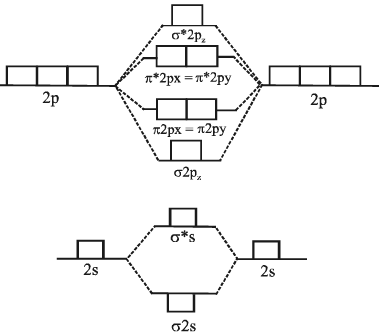M.O Energy level diagram for O2, F2 and Ne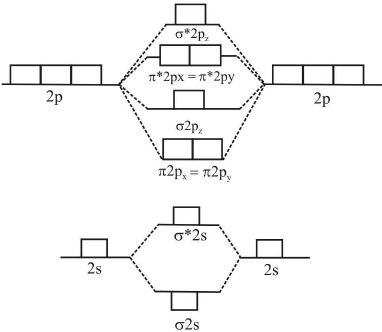M.O energy diagram for Li2, Be2, B2, C2 and N2 molecule

Fajan's Rule:

This rule is used to decide relative ionic & covalent character in a molecule. A molecule is predominantly covalent if

(I) Smaller the size of cation.

(II) larger the size of anion.

(III) greater the charge on cation and anion.

(IV) ion does not have inert gas configuration but it possesses pseudo inert gas configuration (18 electrons in the ultimate shell).

(I)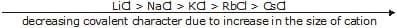(II)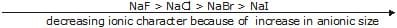(III)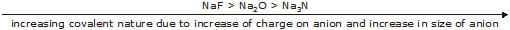(IV) CuCl and NaCl

[Cu ] = [Ar]3d10 ; [Ne ] = [Ne]

Cations with 18-electron shells (pseudo inert gas configuration) has grater polarising power than 8-electron shell (inert gas configuration) ion with the same charge and size. Thus, CuCl is more covalent than NaCl.

Dipole Moment

• If a covalent bond is formed between two dissimilar atoms, eg. A and B, one of the atoms (A or B) must be more electronegative than the other. If A is more electronegative than the shared pair of electrons is drawn near A leaving a positive charge on B and hence making the molecule dipolar (A–B+). The percentage of polar character is given in terms of dipole moment (μ).
• The dipole moment is defined as the product of electric charge q and the distance r between the two atoms of a polar molecule. (μ = e × d) Dipole moment is a vector quantity with direction same as that of the line joining positive and negative centres.
• Thus molecules having dipole moment (μ = 0) are called non polar molecules and molecules with  > 0 are polar. Greater is μ, greater is the polarity.
• For polyatomic molecules with two or more bonds, net dipole moment is the resultant of vector addition of individual moments.
• While expressing dipole moments, generally charge is given in electrostatic units (esu) and distance in angstrom units (1Aº =10–10 m). Thus dipole moment of an electron separated from unit positive charge by a distance 1Aº would be (4.80 × 10–10 esu) × (10-8 cm) = 4.8 × 10–18 esu cm = 4.8 Debye.

Applications of Dipole Moment

1. Dipole moment is helpful in predicting the geometry of the molecule.
2. Dipole moment helps in determining the polarity.
3. Dipole moment can distinguish between symmetrical and non-symmetrical molecules. eg. CO2 has  0 dipole moment as it is symmetrical whereas H2O has a dipole moment of 1.85D.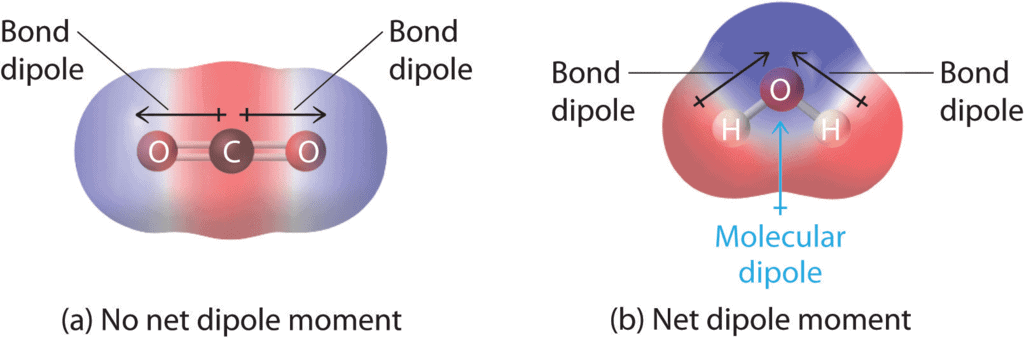1. Cis and trans isomers can be distinguished by dipole moments, usually, cis isomers have higher dipole moment and hence higher polarity e.g.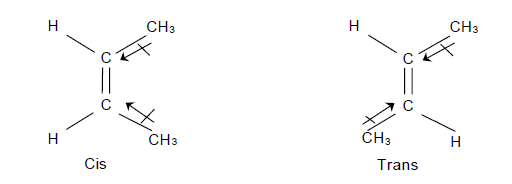1. Dipole moment is greatest for ortho isomer, zero for para isomer and less than that of ortho for meta isomer. o > m > p. e.g.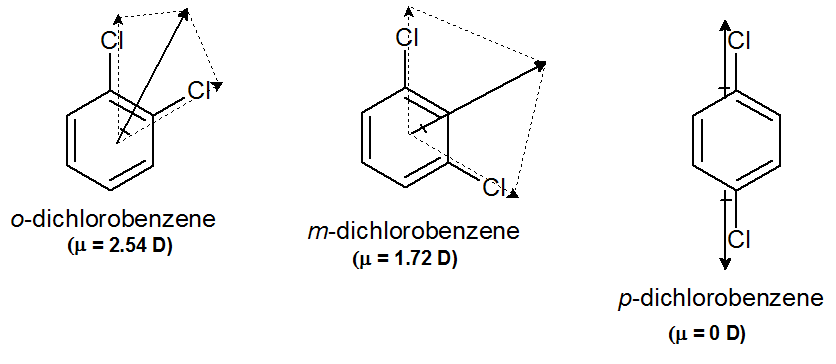1. Ionic character can be determined by using dipole moment

For e.g. experimental dipole moment for HCl is 1.03  and μ = r X eas r = 1.26 and, e = 4.8 × 10-10 esu,μ = 6.05 Debye.Thus the ionic character Thus we can say % ionic character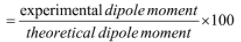1. Hybridisation can be determined by dipole moment for eg.

If a molecule AB2 has μ=0, the s orbitals used  by
A (z < 21) must be sp hybridised e.g. BeF2If a molecule AB3 has μ=0, the s orbitals used by
A (z < 21) must be sp2 hybridised e.g. BF3If a molecule AB4 has μ =0, the s orbitals used by
A (z < 21) must be sp3 hybridised e.g. CCl4

Hydrogen Bonding :

(I) The concept of H-bonding was introduced by Latimer and Rodebush.

(II) H-bonding is said to be formed when slightly acidic hydrogen attached to a strongly electronegative atom such as F, O and N is held with weak electrostatic forces by the non- bonded pair of electron of another atom. That is , it is a dipole-dipole interaction.

(III) Of all the electronegative donor atoms, only F, O. and N enter into stable H-bond formation.

(IV) The weak electrostatic interaction leading to the H-bond formation is shown by dashed(.....) lines.

(V) Greater the electronegativity difference, stronger is the H-bond. For example,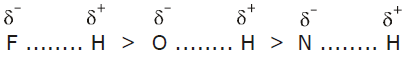Intramolecular H-bonding ;

This type of H-bonding occurs when polar H and electronegative atom are present in the same molecule.

(a) o-hydroxy benzaldehyde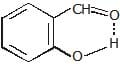(b) o-nitrophenol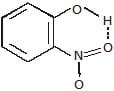Intermolecular H-bonding

This type of H-bonding takes place between hydrogen and electronegative element (like F,O,N) present between molecules of the same substance (H2O and H2O) or different substances (H2O and NH3).

The document Molecular Orbital Theory Notes | Study Chemistry for JEE - JEE is a part of the JEE Course Chemistry for JEE.
All you need of JEE at this link: JEE

## Chemistry for JEE

213 videos|480 docs|369 tests
 Use Code STAYHOME200 and get INR 200 additional OFF

## Chemistry for JEE

213 videos|480 docs|369 tests

Track your progress, build streaks, highlight & save important lessons and more!

,

,

,

,

,

,

,

,

,

,

,

,

,

,

,

,

,

,

,

,

,

;